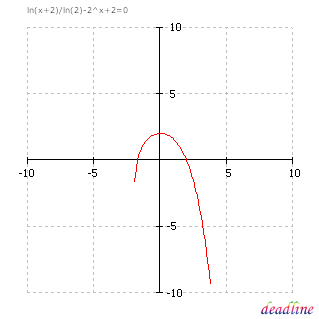﻿ Solve logarithmic equations: ln(x+2)/ln(2)-2^x+2 = 0

# Solve difficult logarithmic equations

The natural logarithm is the logarithm to the base e, where e is approximately equal to 2.71828... (no exact fraction can be given, as e is an irrational number just like pi). The natural logarithm is defined for all positive real numbers. Although this function was not introduced by Napier, it is sometimes known as the Napierian logarithm.

Problem: solve a difficult logarithmic equation, that also involves exponentials

Equation to solve: ln(x+2)/ln(2)-2^x+2 = 0

Interval for roots: [-10 ; 10]

DeadLine can solve logarithmic equations instantly, providing the equation graph and the roots.

Number of roots found: 2

x1 = -1,69009307

x2 = 2

Solve difficult logarithmic equations if you use DeadLine.

Other equations: exponential equations | logarithmic equations | trigonometric equationsDeadLine OnLine - free equation solver. Copyright 2003-2007 Ionut Alex. Chitu. | Contact | Sitemap